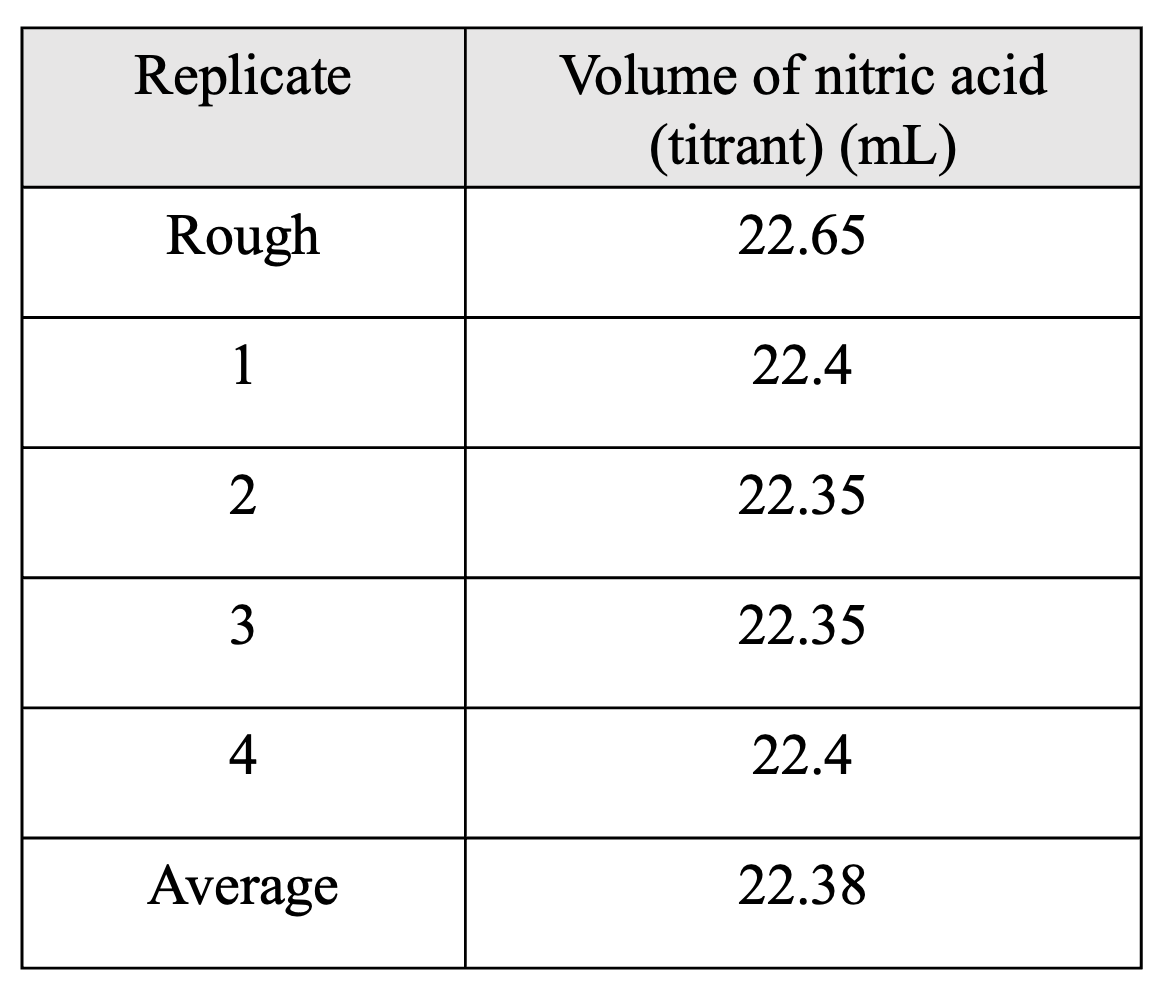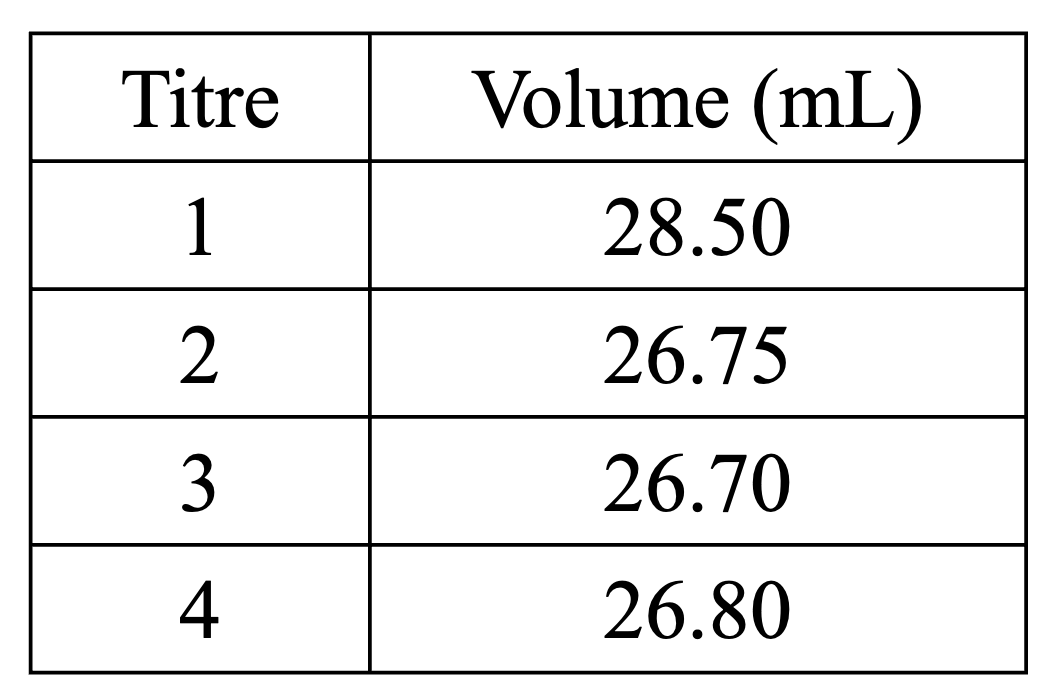# HSC Chemistry – Titration Calculations

This is part of the HSC Chemistry course under the topic Quantitative Analysis.

### HSC Chemistry Syllabus

• Conduct practical investigations to analyse the concentration of an unknown acid or base by titration

### How to do Titration Calculations

This video walks through common examples of calculation problems for titration.

A titration experiment aims to determine the concentration of an unknown solution by using the experimentally determined titre volume.

### What is a Rough Titration?

A rough titration is the first titration trial performed in an experiment. Since this is the first trial, the approximate titre volume required to reach the endpoint (when indicator changes colour) is unknown. As such, it is difficult to know when to decrease the rate at which the titrant is being added to the conical flask, leading to an inaccurate (rough) titre volume being recorded.

The rough titration provides an estimation of the titre volume and helps produce more accurate titrations in subsequent trials. The titre from a rough titration should not be included in the calculation of the concentration of the unknown solution.

### Example 1

A student performs a titration experiment to determine the concentration of a solution of nitric acid. For each trial, 25.0 mL of 0.050 mol/L of a sodium carbonate standard solution is used.

The data from the experiment is shown in the table.Determine the concentration of the nitric acid solution.

The first titre volume (22.65 mL) is derived from a rough titration and should be disregarded when calculating the average titre (22.38 mL)

Step 1: writing a balanced chemical equation

Titration between nitric acid and sodium carbonate is represented by the equation

$$2HNO_3(aq) + Na_2CO_3(aq) \rightarrow Na(NO_3)_2(aq) + H_2O(l) + CO_2(g)$$

Step 2: calculate the number of moles of the standard solution used to reach the endpoint

$$n(Na_2CO_3) = 0.050 \times 0.025 = 0.00125 \hspace{0.1cm} mol$$

Step 3: consider the stoichiometric ratio and calculate the number of moles of the unknown solution

The reaction ratio between nitric acid and sodium carbonate is 2 : 1

$$n(HNO_3) = 0.00125 \times 2 = 0.00250 \hspace{0.1cm} mol$$

Step 4: calculate the concentration of the unknown solution

$$c(HNO_3) = \frac{0.00250}{0.02238}$$

$$c(HNO_3) = 0.11 \hspace{0.1cm} mol \hspace{0.1cm} L^{–1} (2 s.f.)$$

### Example 2

30.0 mL of hydrofluoric acid is titrated with a 0.0350 mol/L sodium carbonate solution.

The result is shown in the table.Determine the concentration of the hydrofluoric acid solution.

In this example, the average titre is not given.

Step 1: calculate the average titre without including the rough titration (28.50 mL).

$$average \hspace{0.1cm} titre = \frac{26.75 + 26.70 + 26.80}{3}$$

$$average \hspace{0.1cm} titre = 26.75 \hspace{0.1cm} mL$$

Step 2: write a balanced chemical equation

$$2HF(aq) + Na_2CO_3(aq) \rightarrow 2NaF(aq) + H_2O(l) + CO_2(g)$$

Step 3: calculate the number of moles of the standard solution

$$n(Na_2CO_3) = 0.0350 \times 0.02675 = 9.36 \times 10^{–4} \hspace{0.1cm} mol$$

Step 4: consider the stoichiometric ratio to calculate the number of moles of the unknown solution

$$n(HF) = 9.36 \times 10^{–4} \times 2 = 1.87 \times 10^{–3} \hspace{0.1cm} mol$$

Step 5: calculate the concentration of the unknown solution

$$c(HF) = \frac{1.87 \times 10^{–3}}{0.030} = 0.0624 \hspace{0.1cm} mol \hspace{0.1cm} L^{–1}$$

### Example 3 – Titration with dilution

A student performs a titration to determine the concentration of a 1.0 L solution of sulfuric acid. 10.0 mL of this solution is transferred to a 100.0 mL volumetric flask and diluted with distilled water. 25.0 mL aliquots of this diluted solution are titrated against a 0.100 mol/L solution of sodium hydrogen carbonate. The average titre volume was 33.40 mL.

What is the concentration of the original sulfuric acid solution?

It is important to note that this titration experiment involves dilution of the unknown solution.

Step 1: write a balanced chemical equation

$$H_2SO_4(aq) + 2NaHCO_3(aq) \rightarrow Na_2SO_4(aq) + 2H_2O(l) + 2CO_2(g)$$

Step 2: calculate the number of moles of the standard solution

$$n(NaHCO_3) = 0.100 \times 0.03340 = 0.003340 \hspace{0.1cm} mol$$

Step 3: consider the stoichiometric ratio to calculate the number of moles of the unknown solution

$$n(H_2SO_4) = \frac{0.003340}{2} = 0.00167 \hspace{0.1cm} mol$$

Step 4: calculate the concentration of the unknown solution

$$c(H_2SO_4)_{diluted} = \frac{0.00167}{0.025} = 0.0668 \hspace{0.1cm} mol \hspace{0.1cm} L^{–1}$$

The original sulfuric acid solution is diluted by a factor of 10 because 10.0 mL was made up to 100.0 mL in the volumetric flask.

Therefore, the original concentration is given by

$$c(H_2SO_4)_{original} = 0.0668 \times 10 = 0.668 \hspace{0.1cm} mol \hspace{0.1cm} L^{–1} (3 s.f.)$$

### Example 4 – Using titration to analyse household substances

Citric acid is a triprotic acid and is found in lemon juice. A student titrated 25.0 mL samples of lemon juice with 0.567 mol/L NaOH. The mean titration volume was 28.50 mL. The molar mass of citric acid is 192.12 g/mol.

What was the concentration of citric acid in the lemon juice? Express your answer in g/L.

Step 1: consider the stoichiometric ratio

Since citric acid (CA) is triprotic, 3 NaOH are required to neutralise every 1 CA. Therefore, the stoichiometric ratio between CA and NaOH is 1 : 3

Step 2: calculate the number of moles of the standard solution

$$n(NaOH) = 0.567 \times 0.02850 = 0.0162 \hspace{0.1cm} mol$$

Step 3: consider the reaction ratio to calculate the number of moles of the unknown solution

$$n(CA) = \frac{0.0162}{3} = 0.00539 \hspace{0.1cm} mol$$

Step 4: calculate the mass of citric acid

$$m(CA) = 0.00539 \times 192.12 = 1.03 \hspace{0.1cm} g$$

Step 5: calculate the concentration in g/L

$$c(CA) = \frac{1.03}{0.025} = 41.4 \hspace{0.1cm} g \hspace{0.1cm} L^{–1}$$